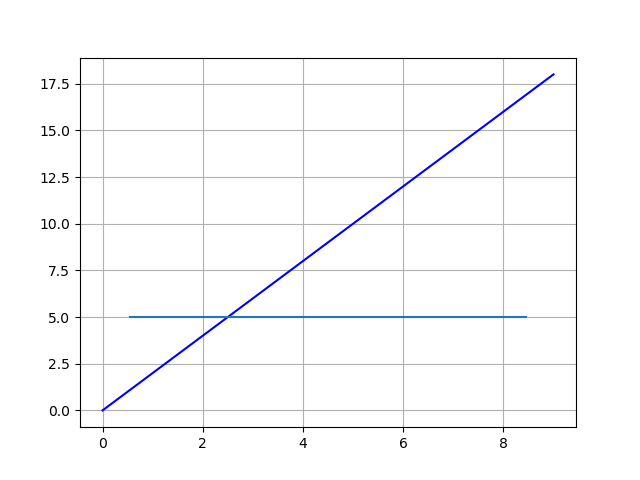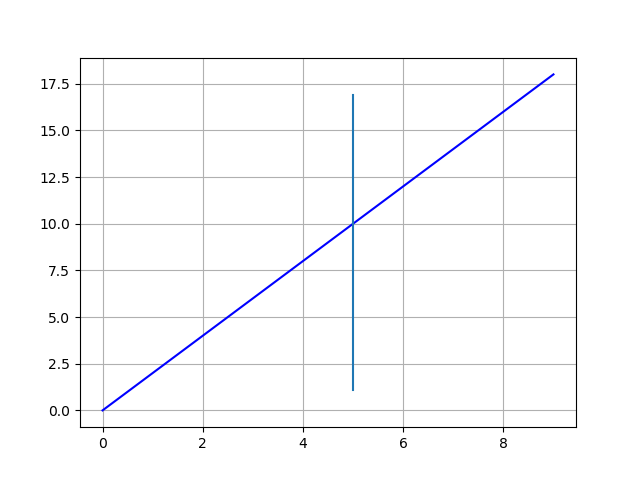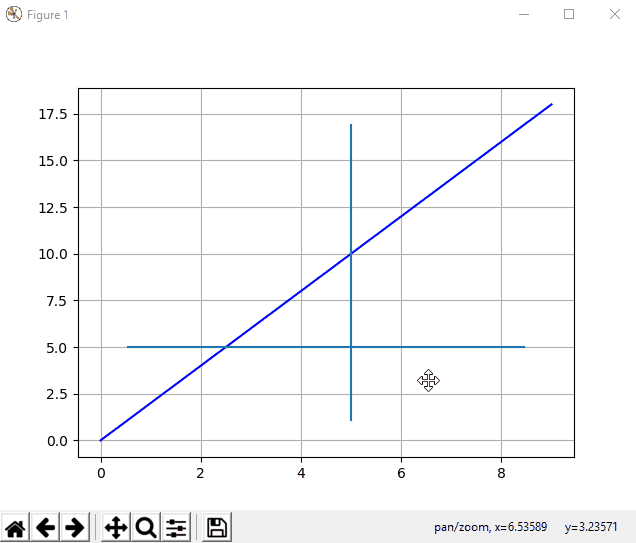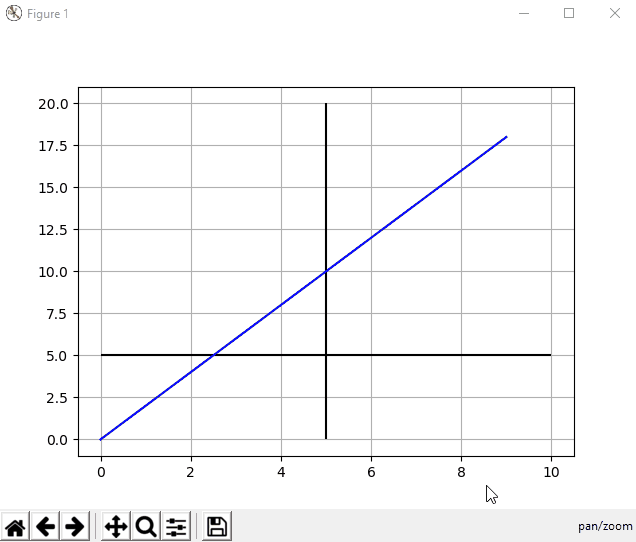# 如何在 Matplotlib 中繪製水平和垂直線

Jinku Hu 2023年1月30日 2019年12月9日

• `axhline()` / `axvline()`
• `hlines()` / `vlines()`

## `axhline` 及 `axvline` 在 Matplotlib 中繪製水平和垂直線

### `axhline` 畫一條水平線

``````matplotlib.pyplot.axhline(y=0, xmin=0, xmax=1, hold=None, **kwargs)
``````

`axhline` 在水平線的 `y` 資料座標中的位置處繪製一條水平線，從 `xmin``xmax`，該點應位於 `0.0` 和之間 `1.0`，其中 `0.0` 是圖的最左側，`1.0` 是圖的最右側。

``````from matplotlib import pyplot as plt

xdata = list(range(10))
ydata = [_*2 for _ in xdata]

plt.plot(xdata, ydata, 'b')

plt.axhline(y=5, xmin=0.1, xmax=0.9)

plt.grid()
plt.show()
``````### `axvline` 畫一條垂直線

``````matplotlib.pyplot.axvline(x=0, ymin=0, ymax=1, hold=None, **kwargs)
``````

`axvline` 在垂直線的 `x` 資料座標中的位置處繪製一條垂直線，從 `ymin``ymax`，該點應在 `0.0` 和之間 `1.0`，其中 `0.0` 是圖的底部，`1.0` 是圖的頂部。

``````from matplotlib import pyplot as plt

xdata = list(range(10))
ydata = [_*2 for _ in xdata]

plt.plot(xdata, ydata, 'b')

plt.axvline(x=5, ymin=0.1, ymax=0.9)

plt.grid()
plt.show()
``````## `hlines` 及 `vlines` 在 Matplotlib 中繪製水平和垂直線

``````hlines(y, xmin, xmax)
``````

``````vlines(x, ymin, ymax)
``````

``````from matplotlib import pyplot as plt

xdata = list(range(10))
ydata = [_*2 for _ in xdata]

plt.plot(xdata, ydata, 'b')

plt.hlines(y=5, xmin=0, xmax=10)
plt.vlines(x=5, ymin=0, ymax=20)

plt.grid()
plt.show()
``````## 在 Matplotlib 中繪製水平和垂直線的結論## Conclusion of drawing horizontal and vertical lines in Matplotlib

If you need the line to be referred to the plot, `axhline` and `axvline` should be the better option.

If you prefer the line to stick to the data coordinate, `hlines` and `vlines` are the better choices.

Author: Jinku Hu

Founder of DelftStack.com. Jinku has worked in the robotics and automotive industries for over 8 years. He sharpened his coding skills when he needed to do the automatic testing, data collection from remote servers and report creation from the endurance test. He is from an electrical/electronics engineering background but has expanded his interest to embedded electronics, embedded programming and front-/back-end programming.